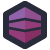Mistakes happen. Errors crop up. Since we know this, we need to defend against it. When it comes to things like account numbers, we can make a rule about which numbers are valid by using a checksum. A simple checksum might be, "Add the digits together, and repeat until you get a single digit, which, after modulus with a constant, must be zero." This means that most simple data-entry errors will result in an invalid account number, but there's still a nice large pool of valid numbers to draw from.

James works for a company that deals with tax certificates, and thus needs to generate numbers which meet a similar checksum rule. Unfortunately for James, this is how his predecessor chose to implement it:

``````while (true)
{
digits = "";
for (int i = 0; i < certificateNumber.ToString().Length; i++)
{
int doubleDigit = Convert.ToInt32(certificateNumber.ToString().Substring(i, 1)) * 2;
digits += (doubleDigit.ToString().Length > 1 ?
Convert.ToInt32(doubleDigit.ToString().Substring(0, 1)) + Convert.ToInt32(doubleDigit.ToString().Substring(1, 1))
: Convert.ToInt32(doubleDigit.ToString().Substring(0, 1)));
}
int result = digits.ToString().Sum(c => c - '0');

if ((result % 10) == 0)
break;
else
certificateNumber++;
}
``````

Which again, gives us one of those pesky numbers. So once we've checked every digit, we'll convert that number back to a useful string, then `Sum` the characters in the string to produce a result. A result which, we hope, is divisible by 10. If not, we check the next number. Repeat and repeat until we get a valid result.
The worst part is, though, is that you can see from the `while` loop that this is just dropped into a larger method. This isn't a single function which generates valid certificate numbers. This is a block that gets dropped in line. Similar, but slightly different blocks are dropped in when numbers need to be validated. There's no single `isValidCertificate` method.[Advertisement] Continuously monitor your servers for configuration changes, and report when there's configuration drift. Get started with Otter today!